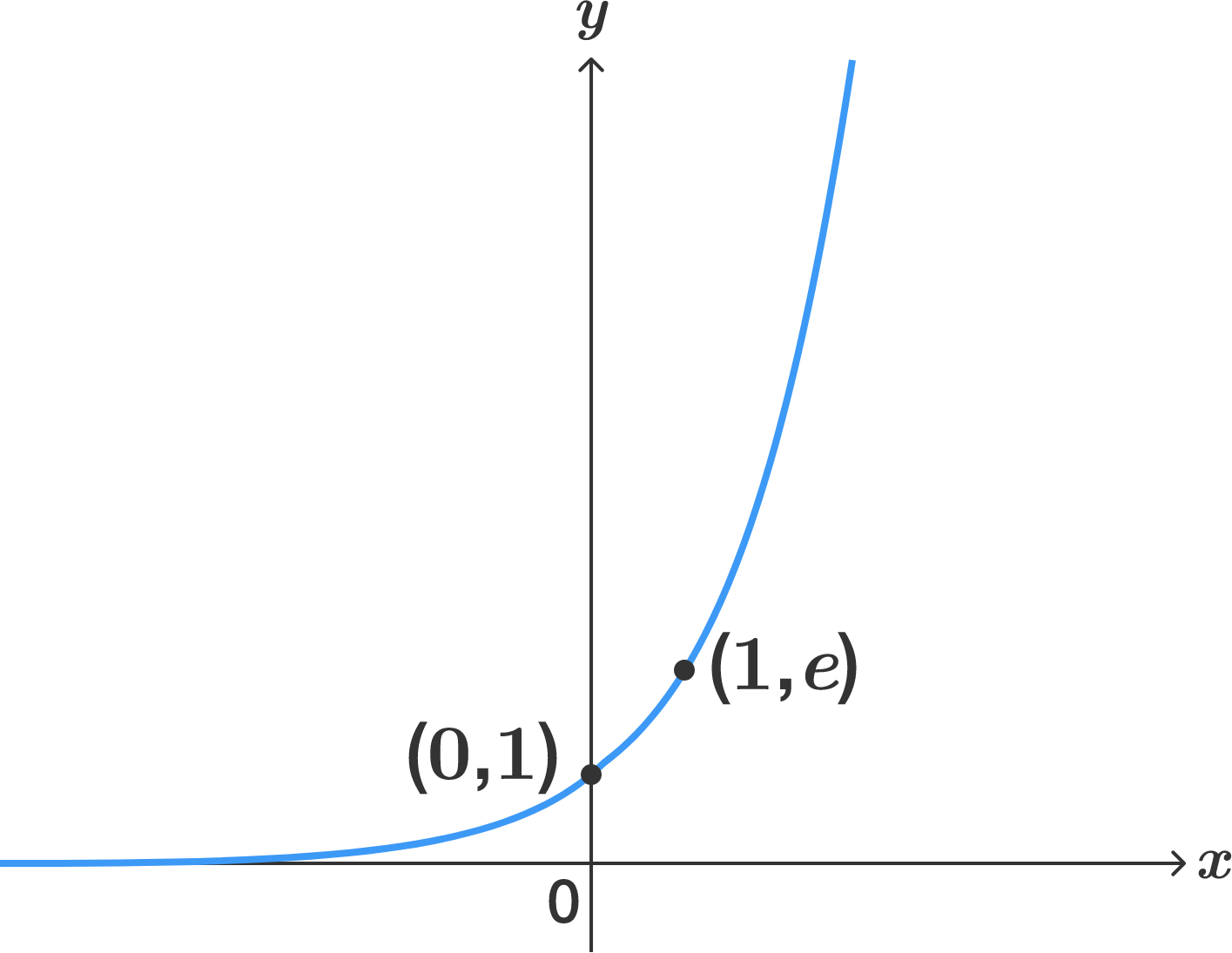# One-one

Calculus Level 2

We know that the function $f(x)=e^x$ is injective on $R$ and that its derivative is always positive at all points.

Is it true that any function whose derivative vanishes at least at one point is many-to-one on the interval that contains those points?×